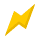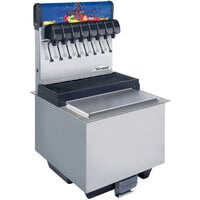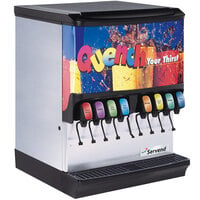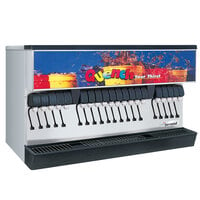120 Volts\$4,789.00/Each120 Volts\$1,939.00/Each120 Volts\$2,559.00/Each120 Volts\$3,279.00/Each120 Volts\$4,739.00/Each

Notify me when this
product is back in stock:120 Volts\$9,069.00/Each120 Volts\$7,999.00/Each120 Volts\$2,879.00/Each120 Volts\$6,319.00/Each

\$3,089.00/Each

\$5,039.00/Each

\$9,069.00/Each

\$10,689.00/Each

\$14,339.00/Each

\$11,699.00/Each

\$13,369.00/Each120 Volts\$6,249.00/Each